# C QQ and PP Plots

https://homepage.divms.uiowa.edu/~luke/classes/STAT4580/qqpp.html

## C.1 QQ Plot

One way to assess how well a particular theoretical model describes a data distribution is to plot data quantiles against theoretical quantiles.

Base graphics provides qqnorm, lattice has qqmath, and ggplot2 has geom_qq.

The default theoretical distribution used in these is a standard normal, but, except for qqnorm, these allow you to specify an alternative.

For a large sample from the theoretical distribution the plot should be a straight line through the origin with slope 1:

library(ggplot2)

n <- 10000
ggplot() + geom_qq(aes(sample = rnorm(n)))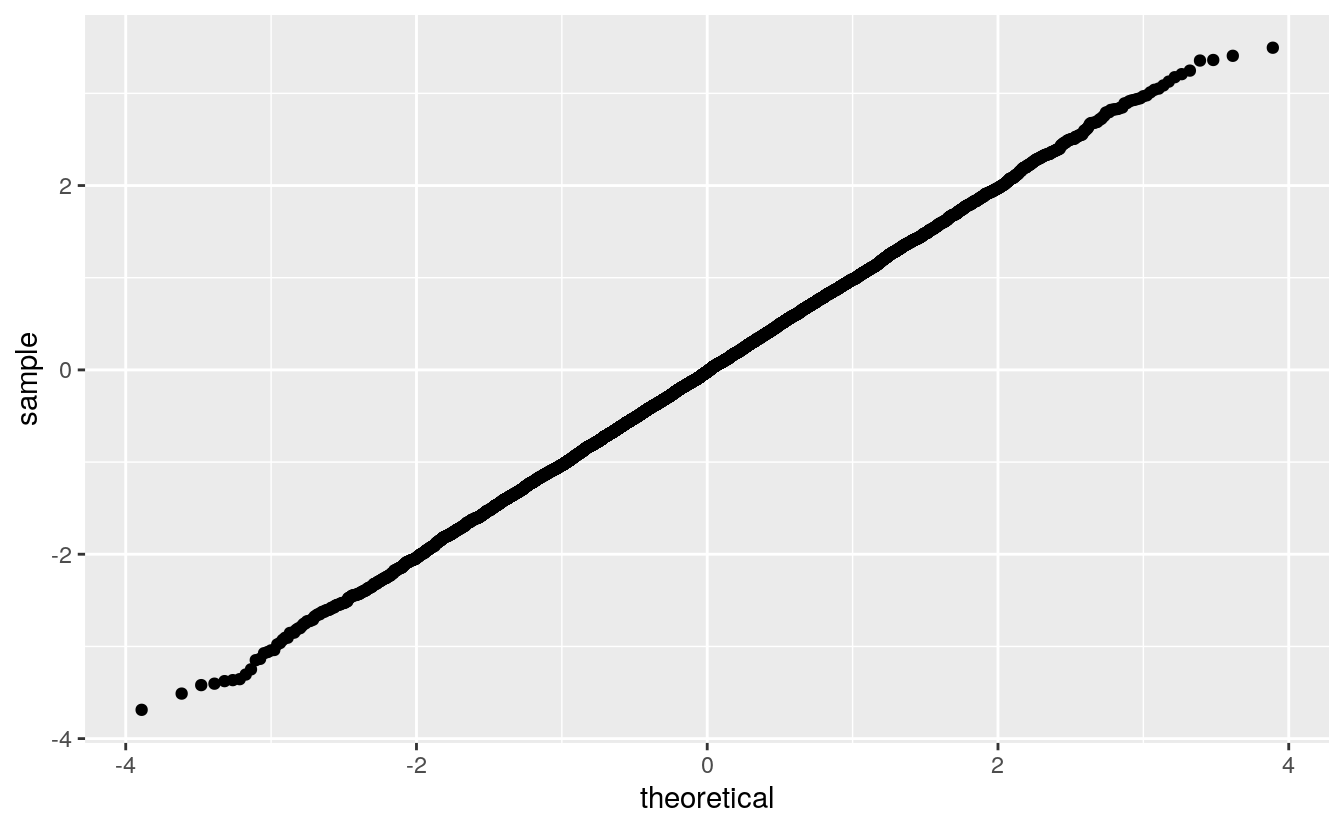If the plot is a straight line with a different slope or intercept, then the data distribution corresponds to a location-scale transformation of the theoretical distribution.

The slope is the scale and the intercept is the location:

ggplot() +
geom_qq(aes(sample = rnorm(n, 10, 4))) +
geom_abline(intercept = 10, slope = 4,
color = "red", size = 1.5, alpha = 0.8)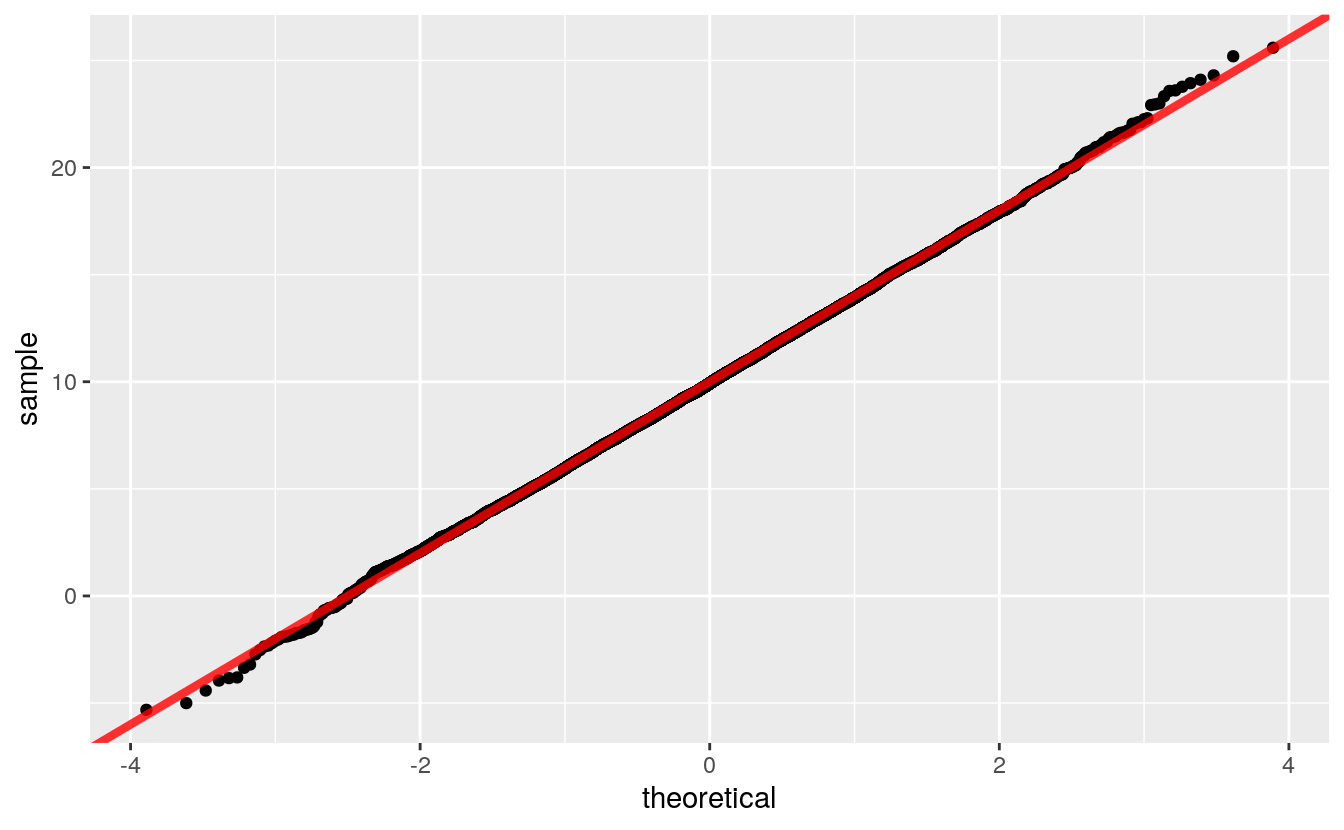The QQ plot can be constructed directly as a scatterplot of the sorted sample $$i = 1, \dots, n$$ against quantiles for

$p_i = \frac{i}{n} - \frac{1}{2n}$

p <- (1 : n) / n - 0.5 / n
y <- rnorm(n, 10, 4)
ggplot() + geom_point(aes(x = qnorm(p), y = sort(y)))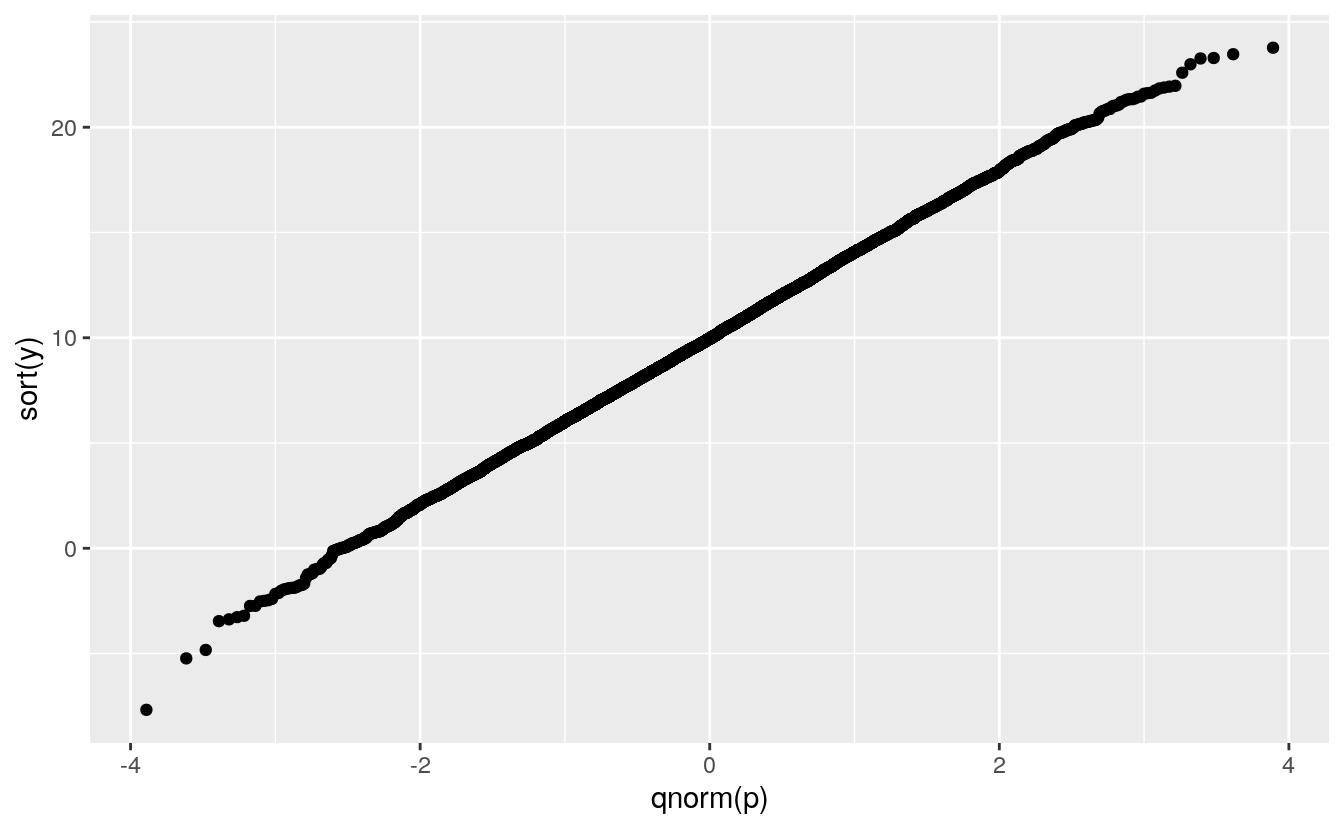## C.2 Some Examples

The histograms and density estimates for the duration variable in the geyser data set showed that the distribution is far from a normal distribution, and the normal QQ plot shows this as well:

library(MASS)
ggplot(geyser) + geom_qq(aes(sample = duration))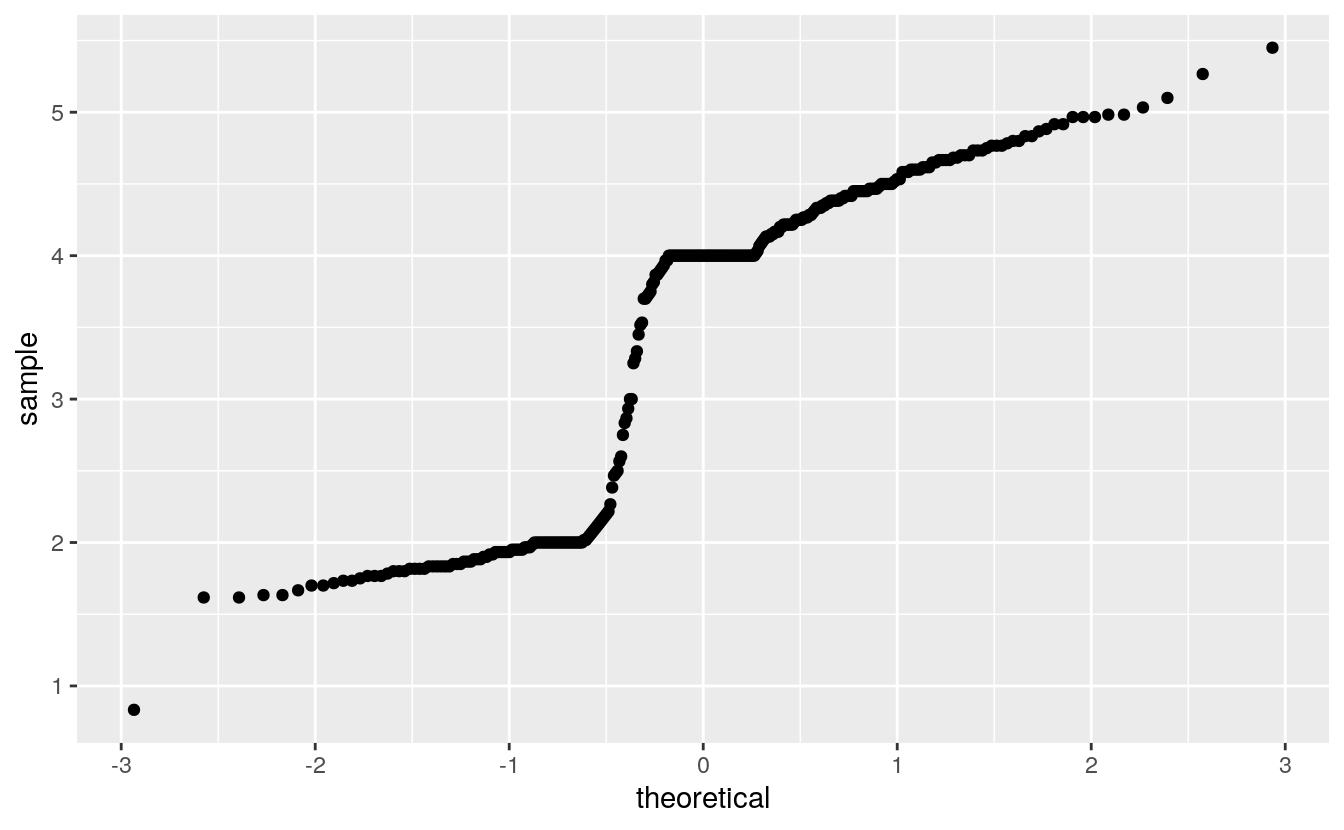Except for rounding the parent heights in the Galton data seemed not too fat from normally distributed:

library(psych)
library(UsingR)

ggplot(galton) + geom_qq(aes(sample = parent))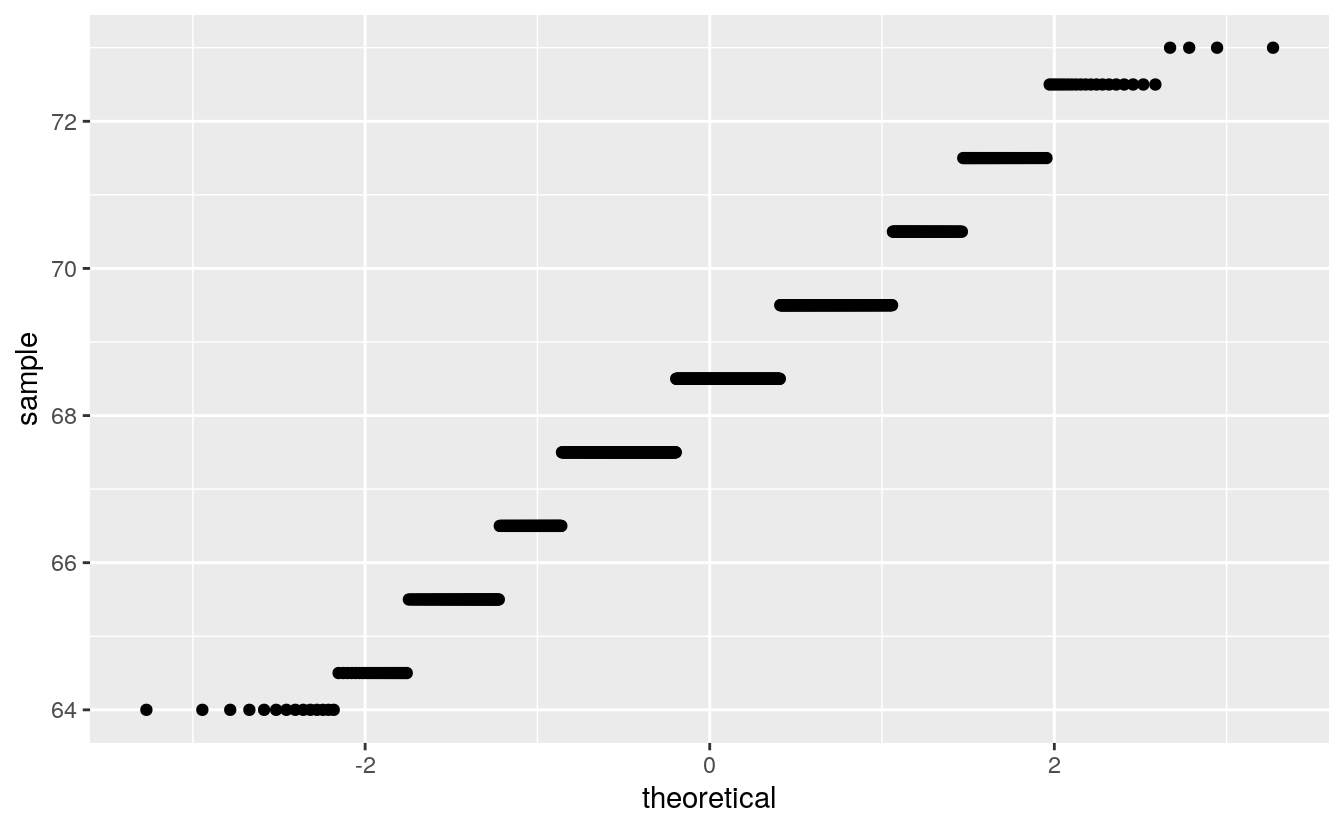Rounding interferes more with this visualization than with a histogram or a density plot.

Rounding is more visible with this visualization than with a histogram or a density plot.

Another Gatlton dataset available in the UsingR package with less rounding is father.son:

library(UsingR)
ggplot(father.son) + geom_qq(aes(sample = fheight))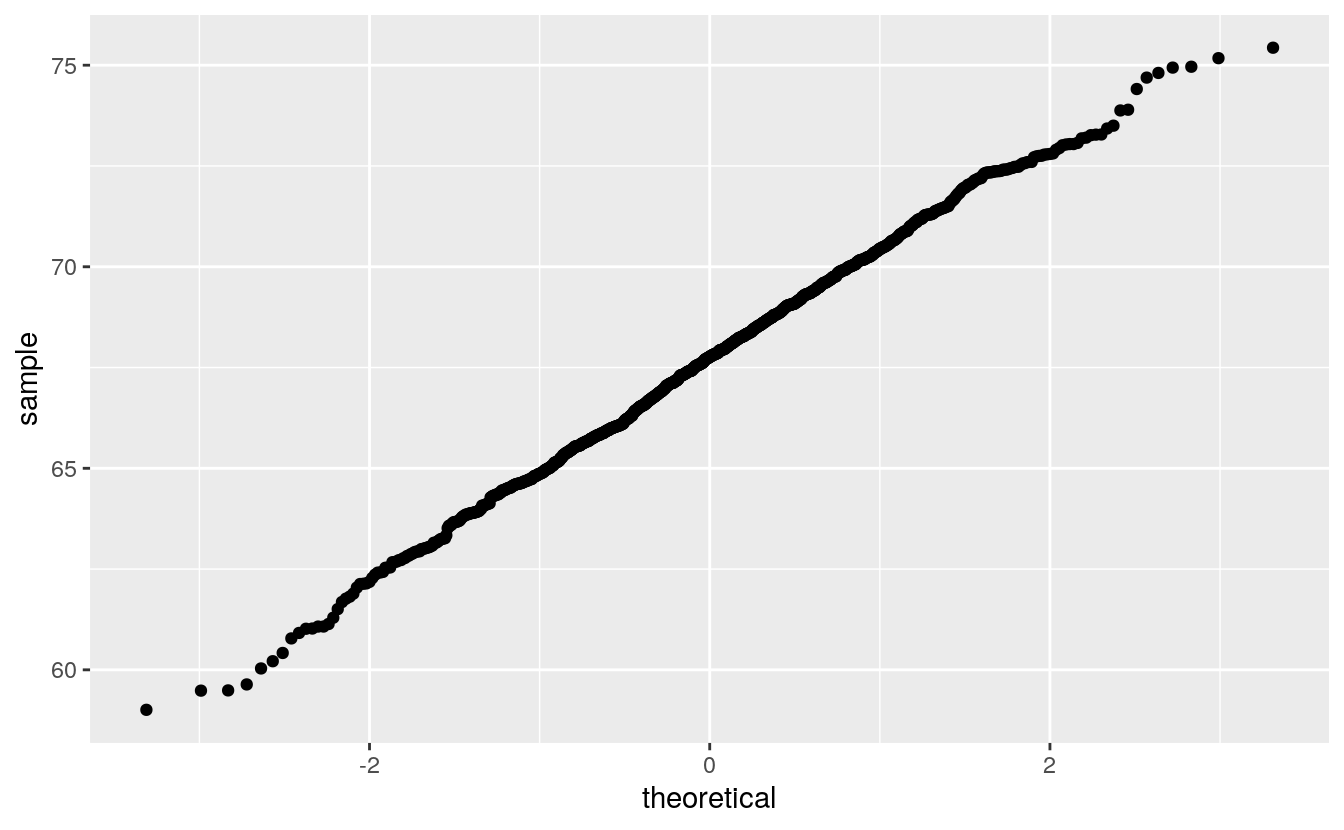The middle seems to be fairly straight, but the ends are somewhat wiggly.

How can you calibrate your judgment?

## C.3 Calibrating the Variability

One approach is to use simulation, sometimes called a graphical bootstrap.

The nboot function will simulate R samples from a normal distribution that match a variable x on sample size, sample mean, and sample SD.

The result is returned in a dataframe suitable for plotting:

nsim <- function(n, m = 0, s = 1) {
z <- rnorm(n)
m + s * ((z - mean(z)) / sd(z))
}

nboot <- function(x, R) {
n <- length(x)
m <- mean(x)
s <- sd(x)
do.call(rbind,
lapply(1 : R,
function(i) {
xx <- sort(nsim(n, m, s))
p <- seq_along(x) / n - 0.5 / n
data.frame(x = xx, p = p, sim = i)
}))
}

Plotting these as lines shows the variability in shapes we can expect when sampling from the theoretical normal distribution:

gb <- nboot(father.son$fheight, 50) tibble::as_tibble(gb) #> # A tibble: 53,900 x 3 #> x p sim #> <dbl> <dbl> <int> #> 1 59.8 0.000464 1 #> 2 59.9 0.00139 1 #> 3 59.9 0.00232 1 #> 4 60.8 0.00325 1 #> 5 60.8 0.00417 1 #> 6 60.9 0.00510 1 #> # … with 53,894 more rows ggplot() + geom_line(aes(x = qnorm(p), y = x, group = sim), color = "gray", data = gb)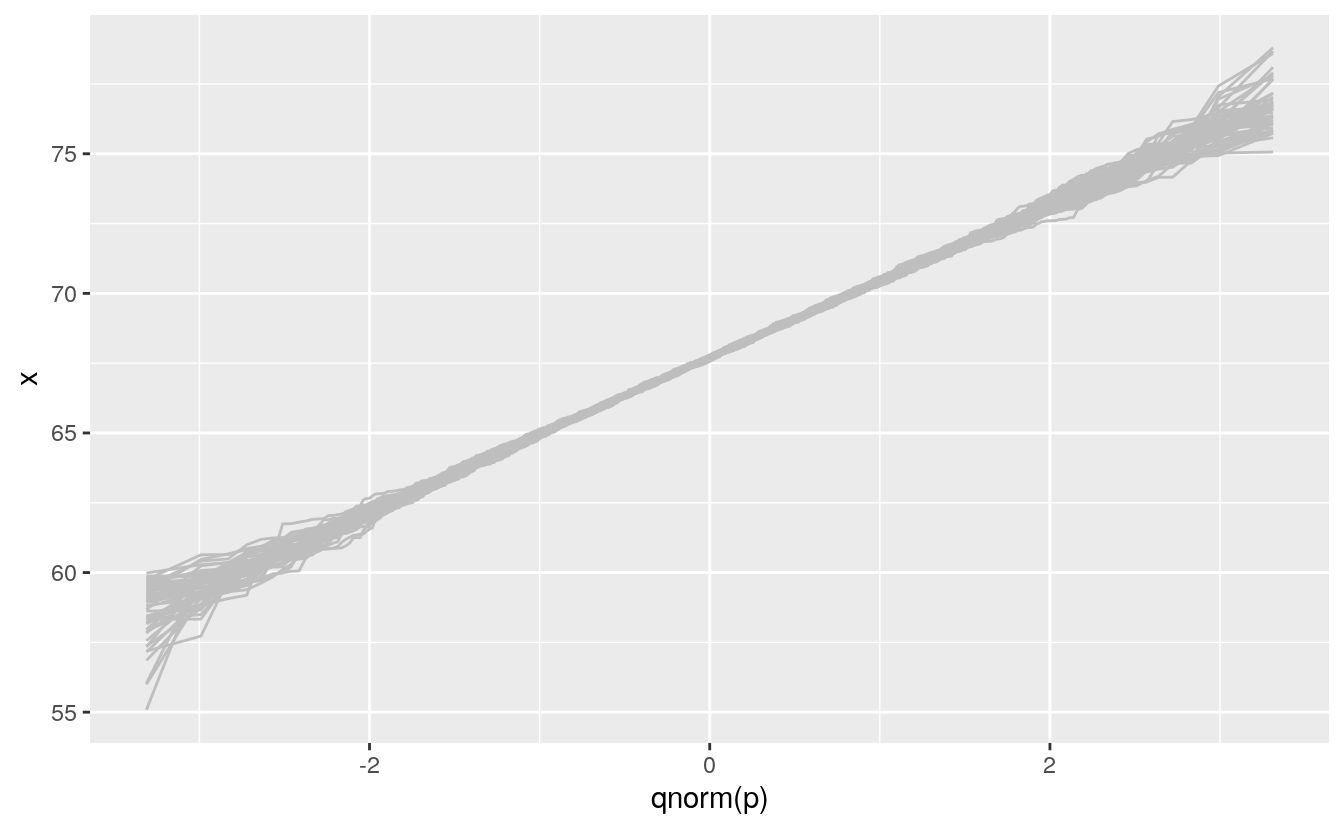We can then insert this simulation behind our data to help calibrate the visualization: ggplot(father.son) + geom_line(aes(x = qnorm(p), y = x, group = sim), color = "gray", data = gb) + geom_qq(aes(sample = fheight))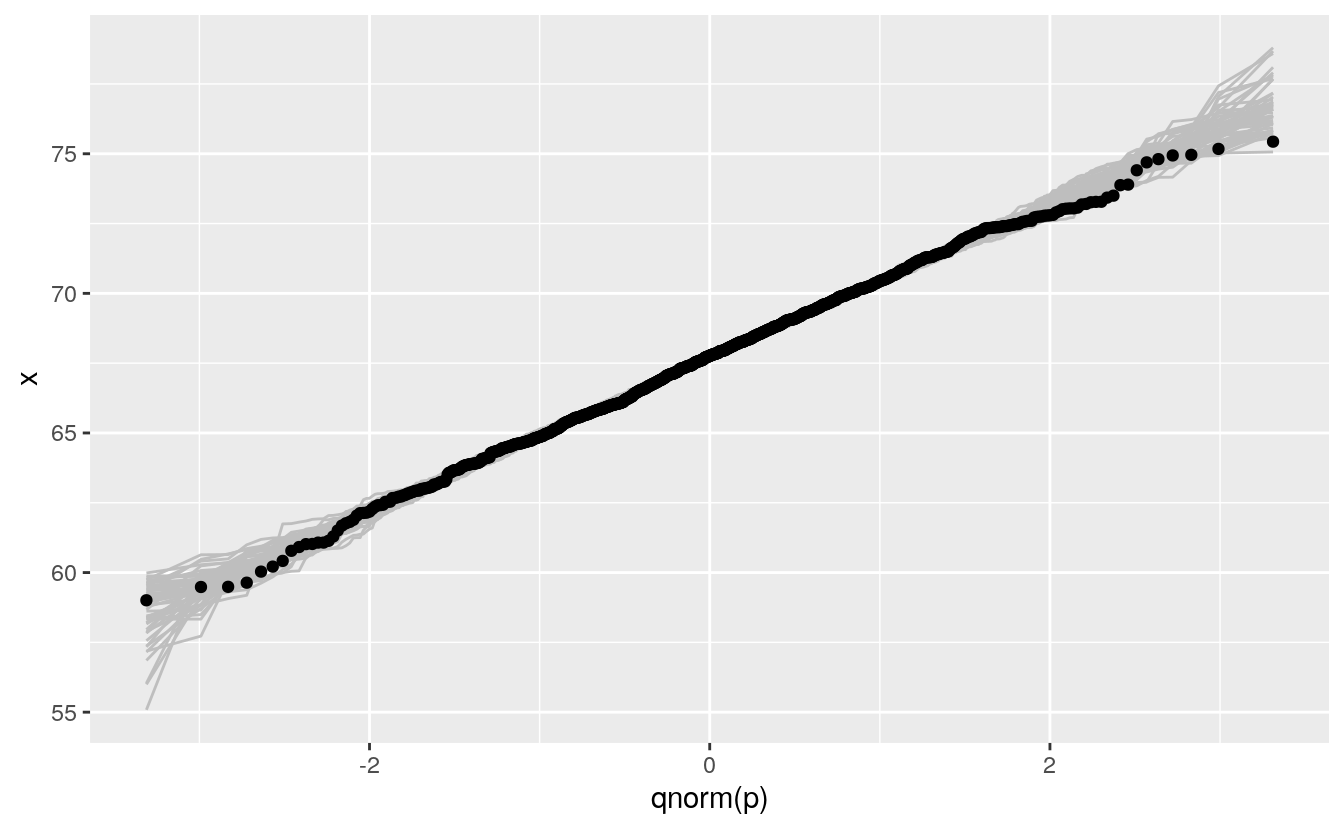## C.4 Scalability For large sample sizes overplotting will occur: ggplot(diamonds) + geom_qq(aes(sample = price))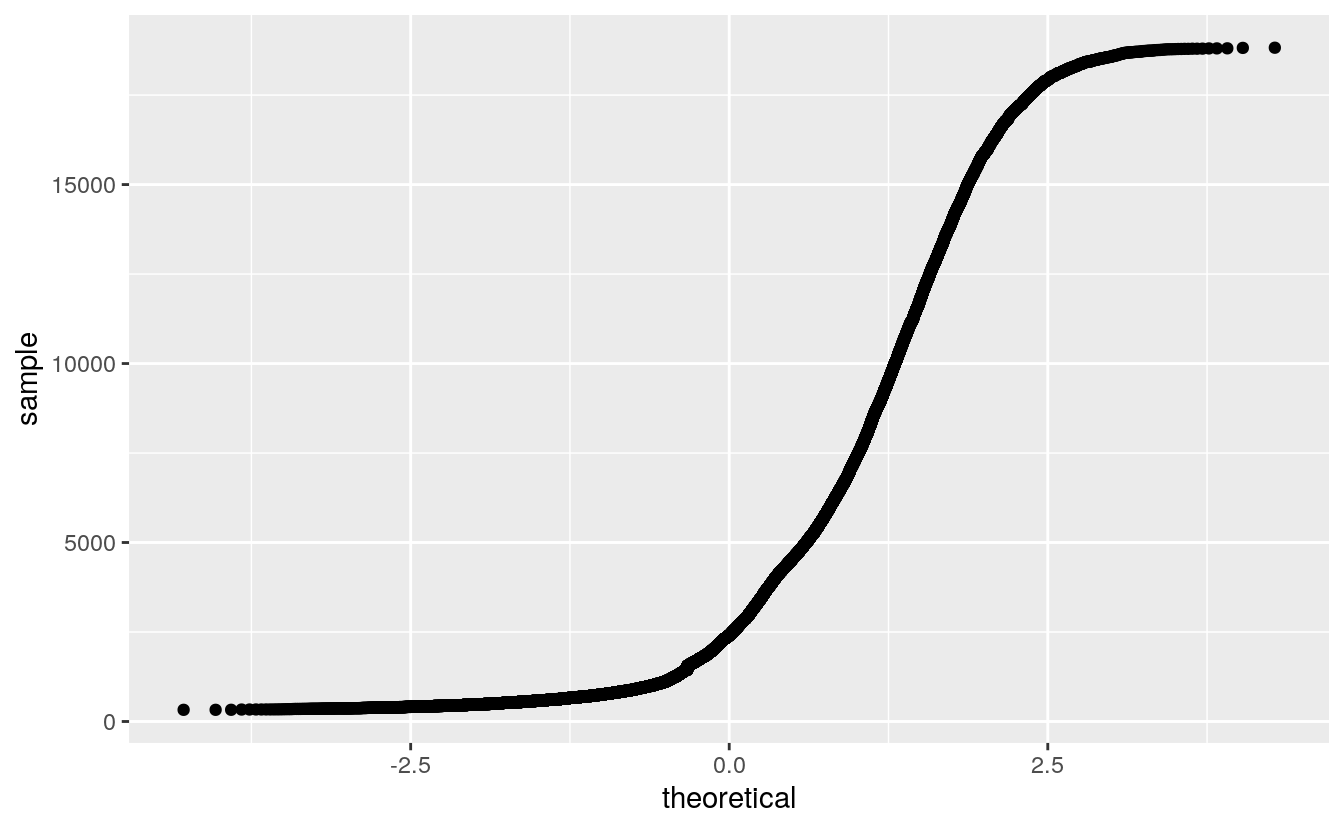This can be alleviated by using a grid of quantiles: nq <- 100 p <- (1 : nq) / nq - 0.5 / nq ggplot() + geom_point(aes(x = qnorm(p), y = quantile(diamonds$price, p)))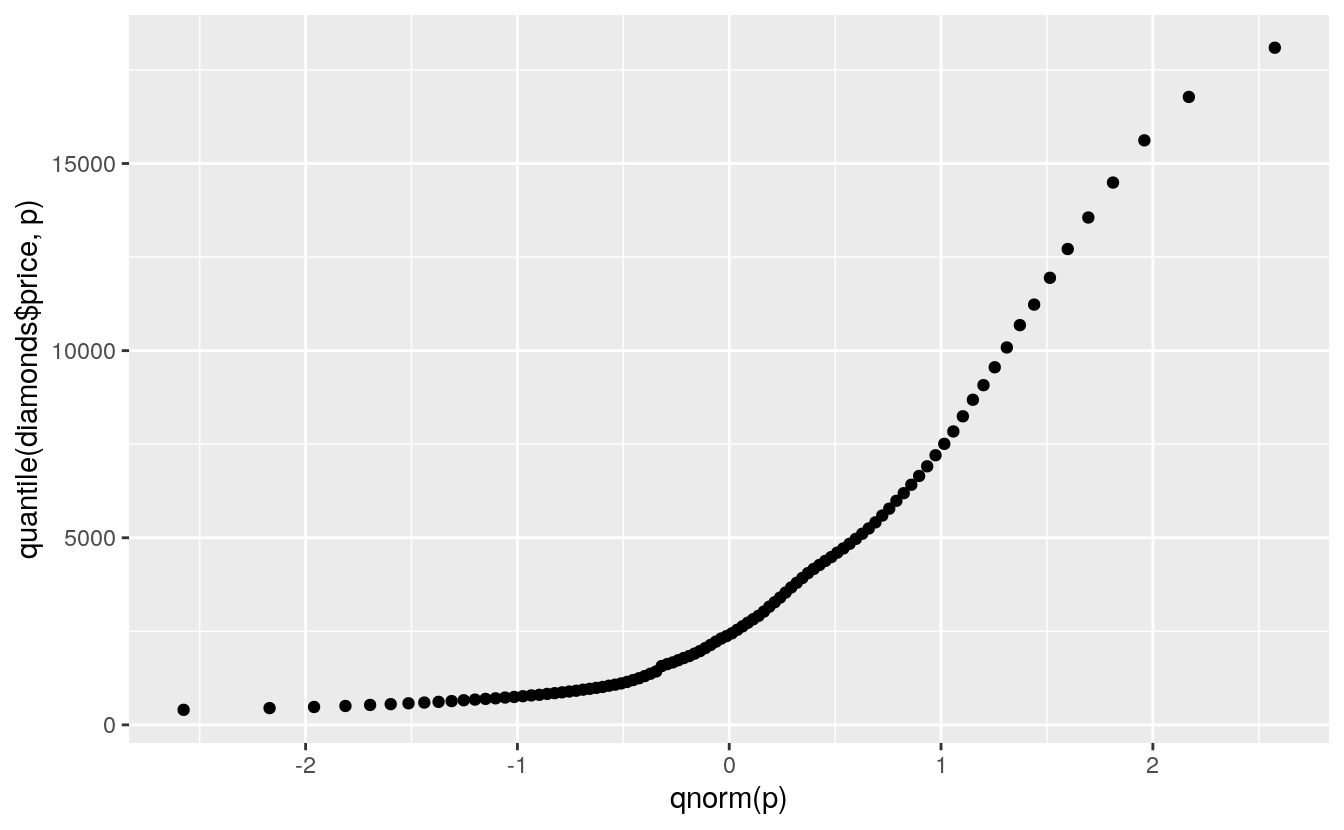A more reasonable model might be an exponential distribution:

wf <- faithful$waiting ggplot() + geom_point(aes(x = quantile(wg, p), y = quantile(wf, p)))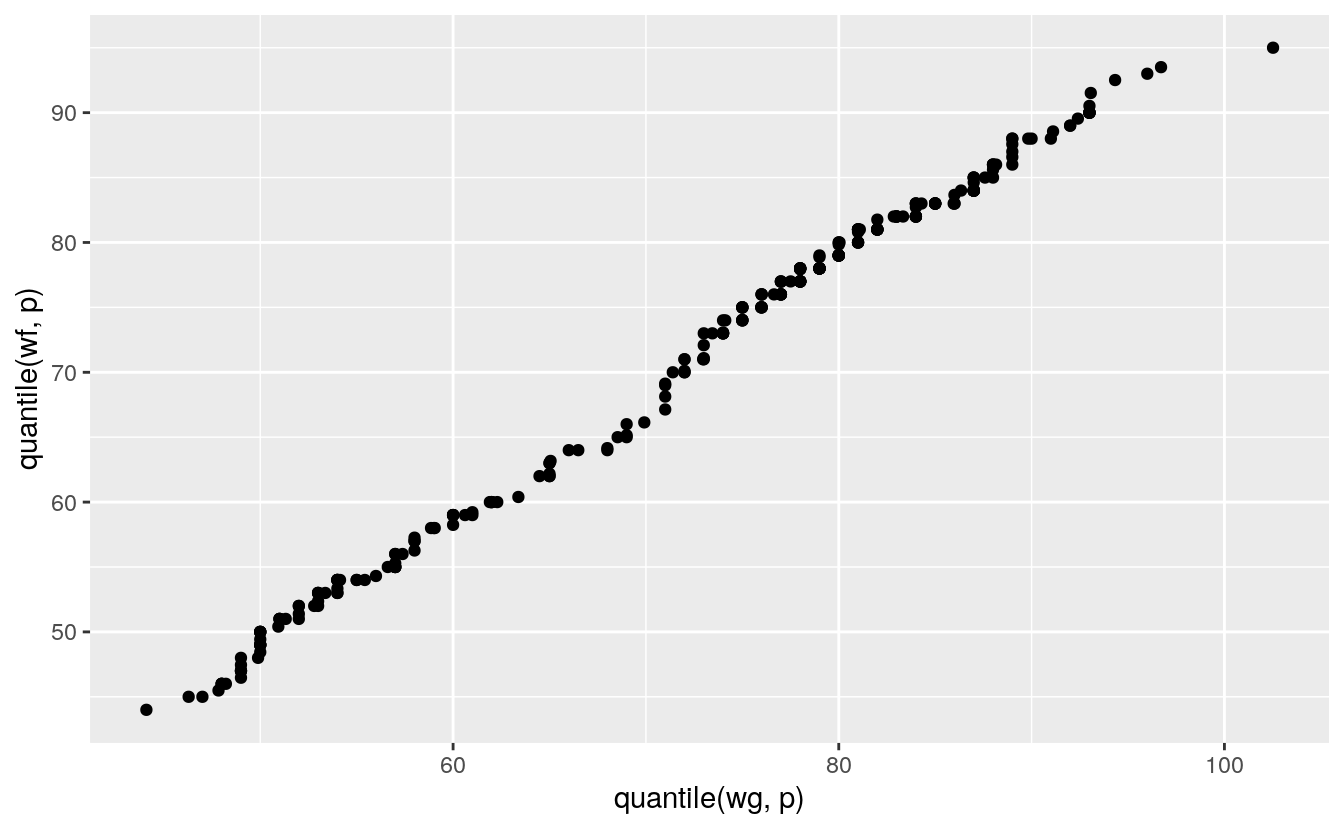## C.6 PP Plots The PP plot for comparing a sample to a theoretical model plots the theoretical proportion less than or equal to each observed value against the actual proportion. For a theoretical cumulative distribution function F this means plotting $F(x(i))∼pi$ For the fheight variable in the father.son data: m <- mean(father.son$fheight)
s <- sd(father.son\$fheight)
n <- nrow(father.son)
p <- (1 : n) / n - 0.5 / n
ggplot(father.son) + geom_point(aes(x = p, y = sort(pnorm(fheight, m, s))))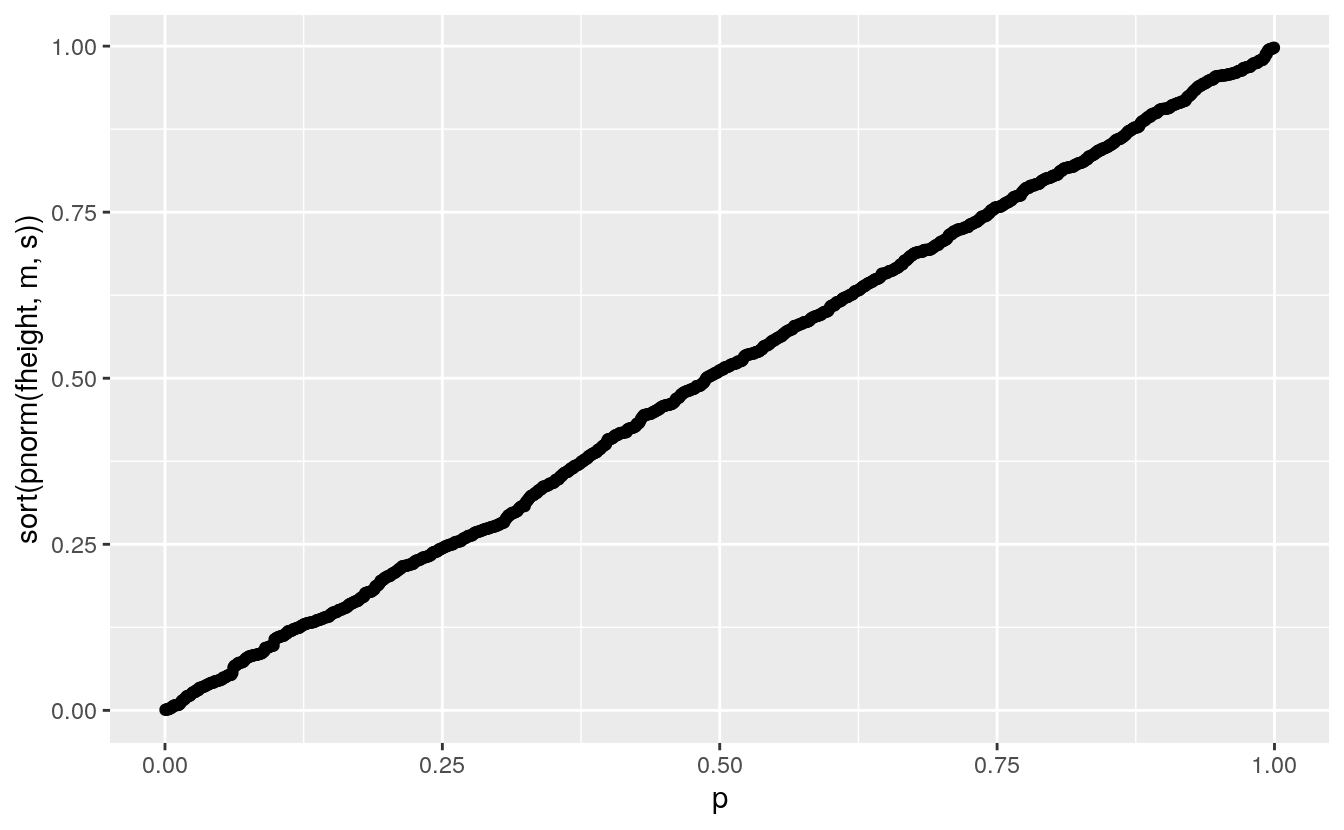• The values on the vertical axis are the probability integral transform of the data for the theoretical distribution.

• If the data are a sample from the theoretical distribution then these transforms would be uniformly distributed on [0,1].

• The PP plot is a QQ plot of these transformed values against a uniform distribution.

• The PP plot goes through the points (0,0) and (1,1) and so is much less variable in the tails:

pp <- ggplot() +
geom_line(aes(x = p, y = pnorm(x, m, s), group = sim),
color = "gray", data = gb)
pp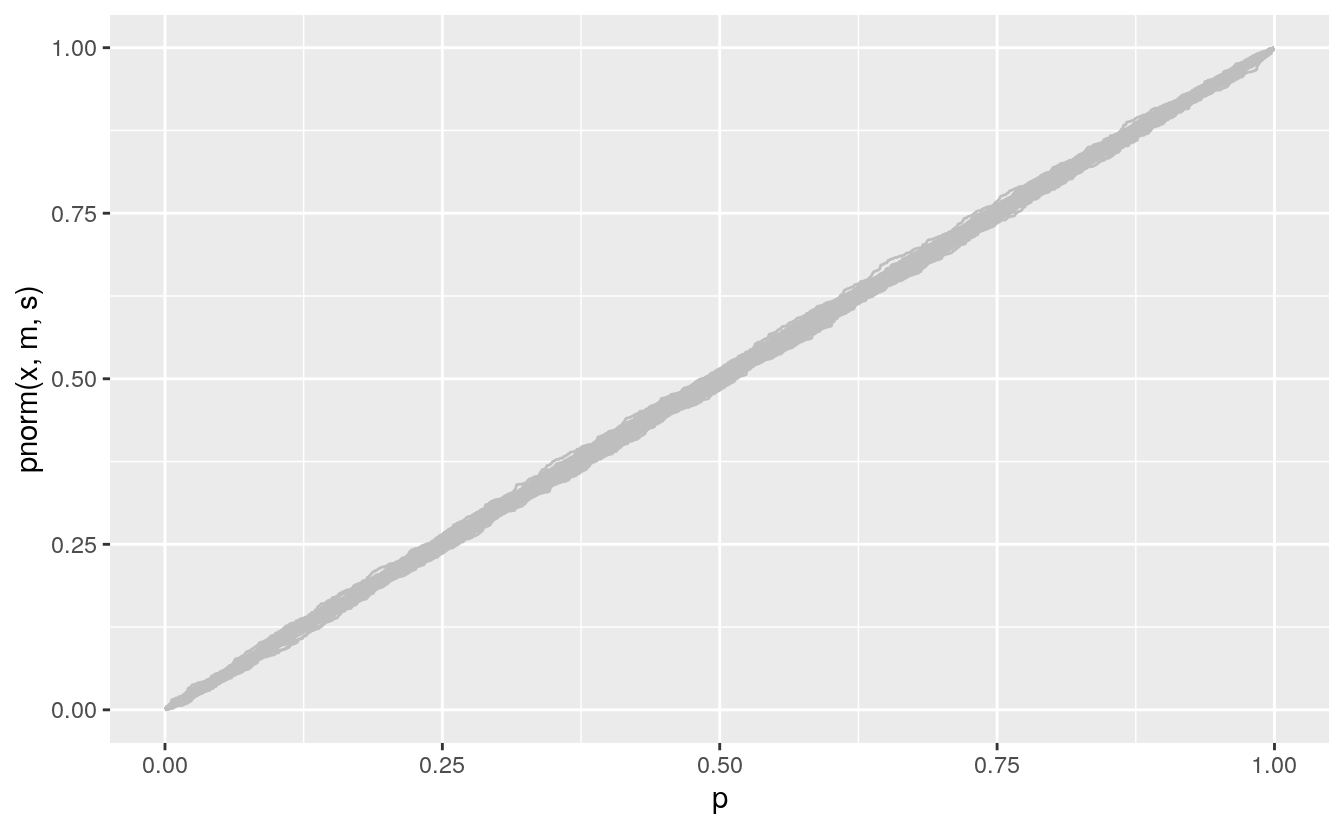pp +
geom_point(aes(x = p, y = sort(pnorm(fheight, m, s))), data = (father.son))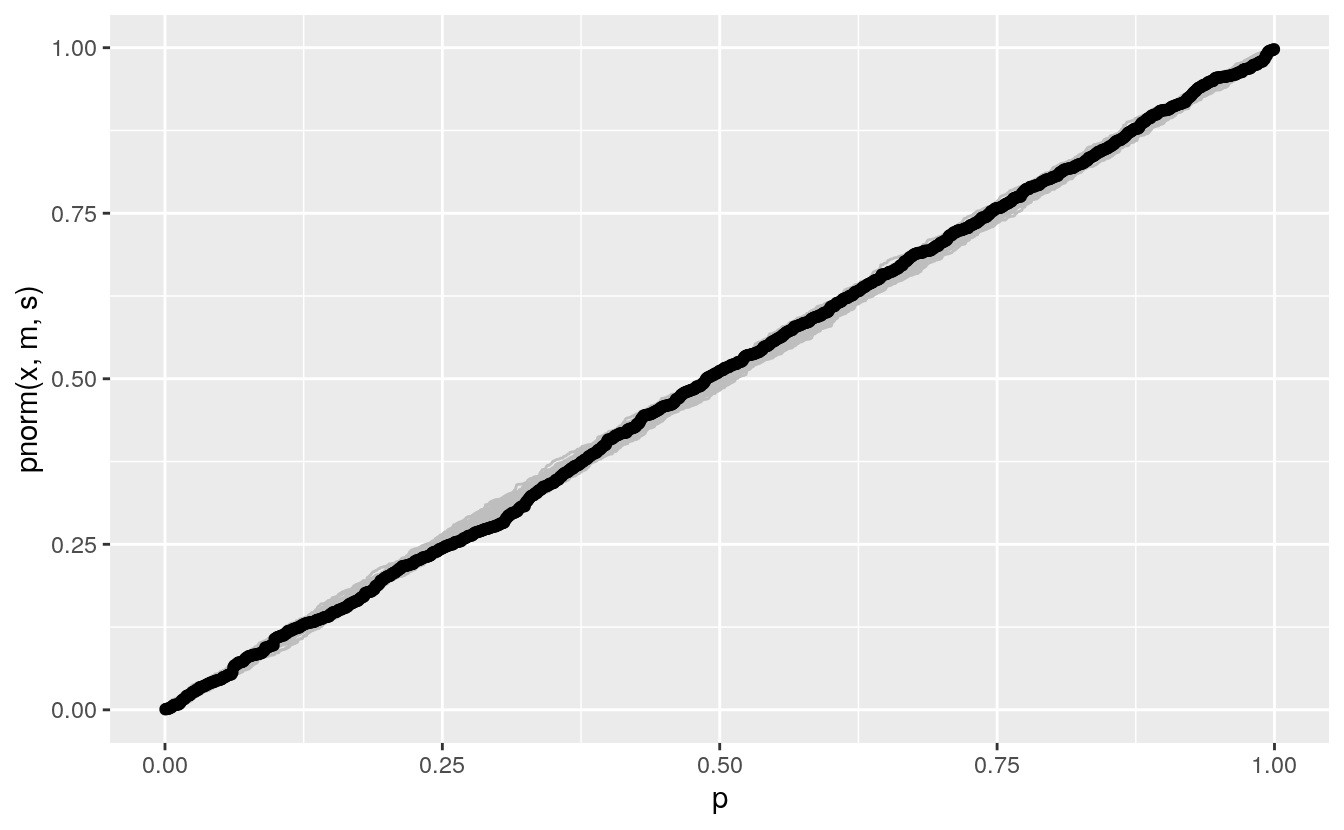The PP plot is also less sensitive to deviations in the tails.

A compromise between the QQ and PP plots uses the arcsine square root variance-stabilizing transformation, which makes the variability approximately constant across the range of the plot:

vpp <- ggplot() +
geom_line(aes(x = asin(sqrt(p)), y = asin(sqrt(pnorm(x, m, s))), group = sim),
color = "gray", data = gb)
vpp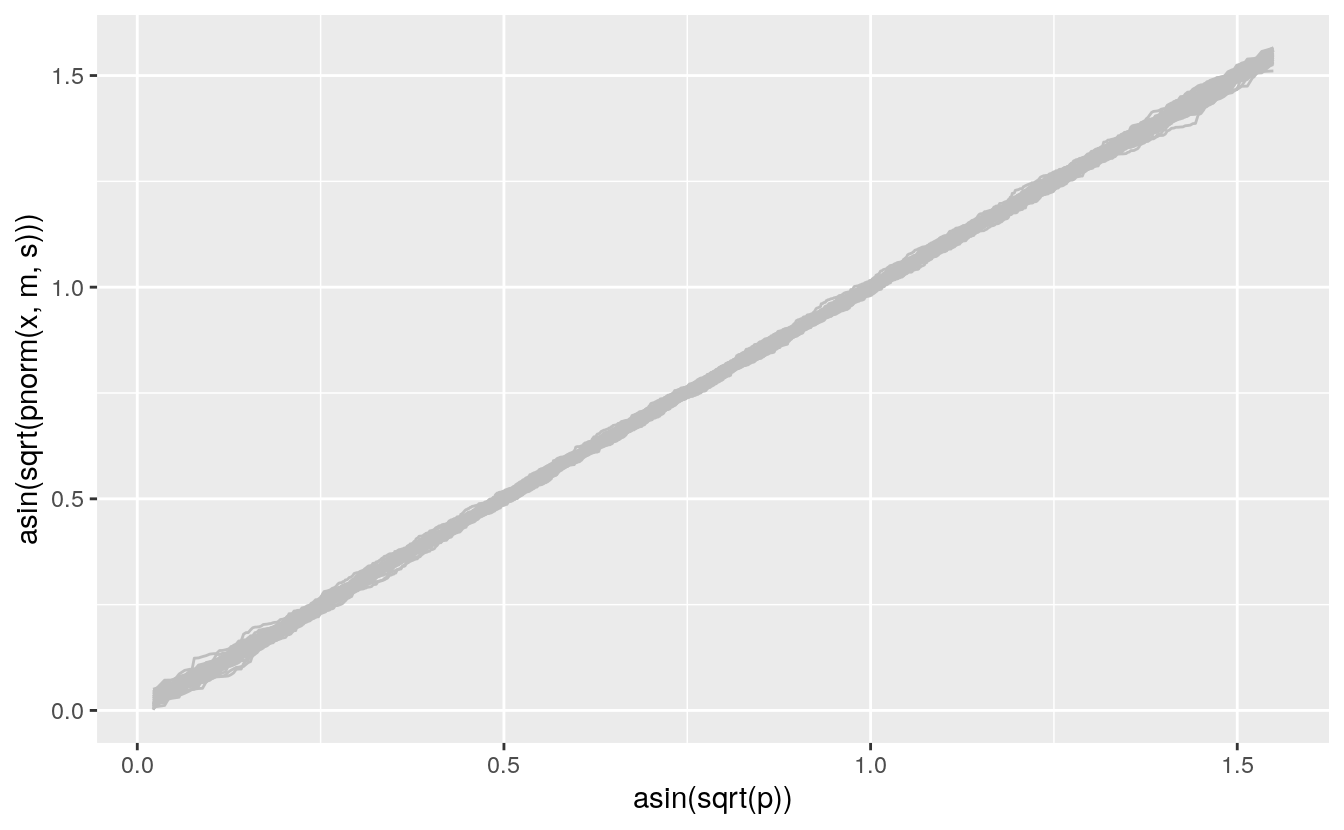vpp +
geom_point(aes(x = asin(sqrt(p)), y = sort(asin(sqrt(pnorm(fheight, m, s))))),
data = (father.son))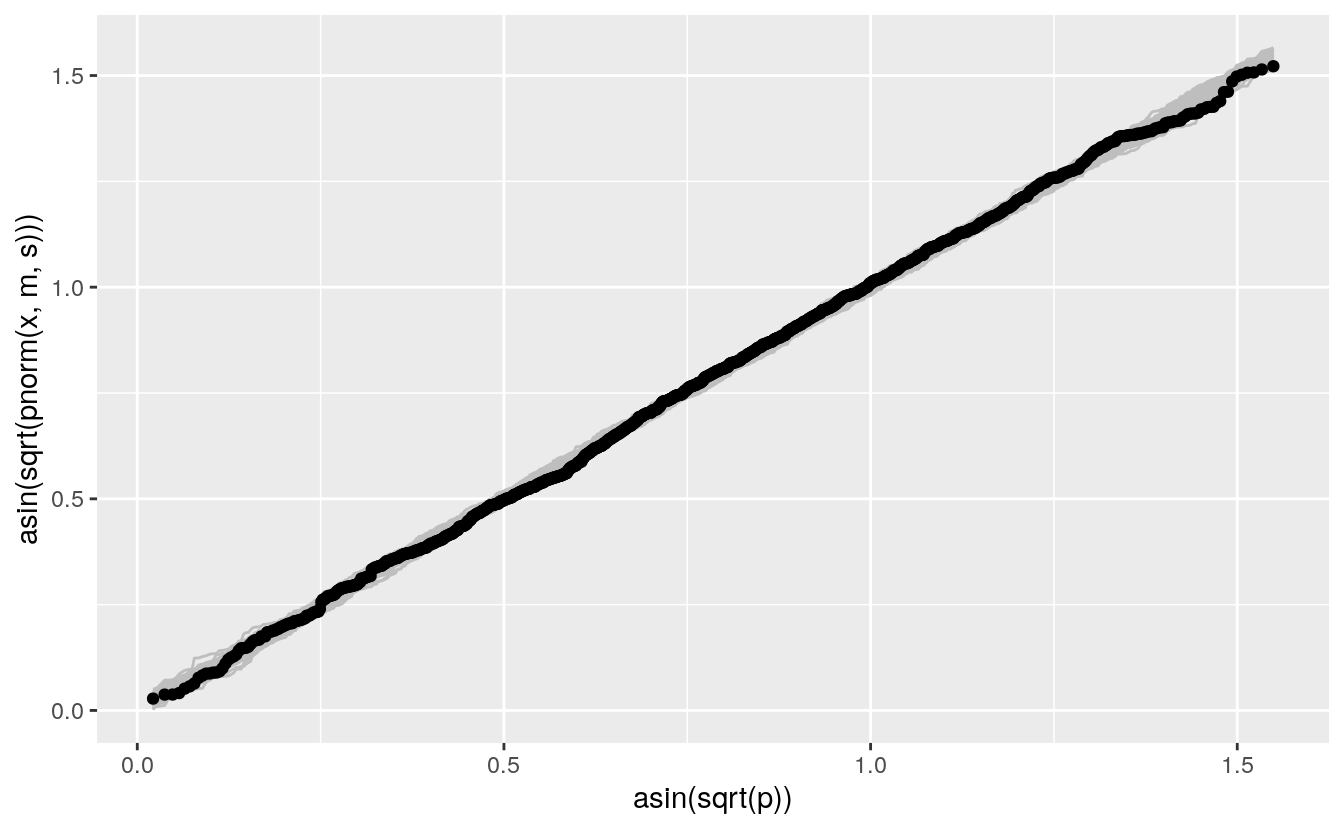## C.7 Plots For Assessing Model Fit

• Both QQ and PP plots can be used to asses how well a theoretical family of models fits your data, or your residuals.

• To use a PP plot you have to estimate the parameters first.

• For a location-scale family, like the normal distribution family, you can use a QQ plot with a standard member of the family.

• Some other families can use other transformations that lead to straight lines for family members:

The Weibull family is widely used in reliability modeling; its CDF is $F(t) = 1 - \exp\left\{-\left(\frac{t}{b}\right)^a\right\}$

• The logarithms of Weibull random variables form a location-scale family.

• Special paper used to be available for Weibull probability plots.

A Weibull QQ plot for price in the diamonds data:

n <- nrow(diamonds)
p <- (1 : n) / n - 0.5 / n
ggplot(diamonds) +
geom_point(aes(x = log10(qweibull(p, 1, 1)), y = log10(sort(price))))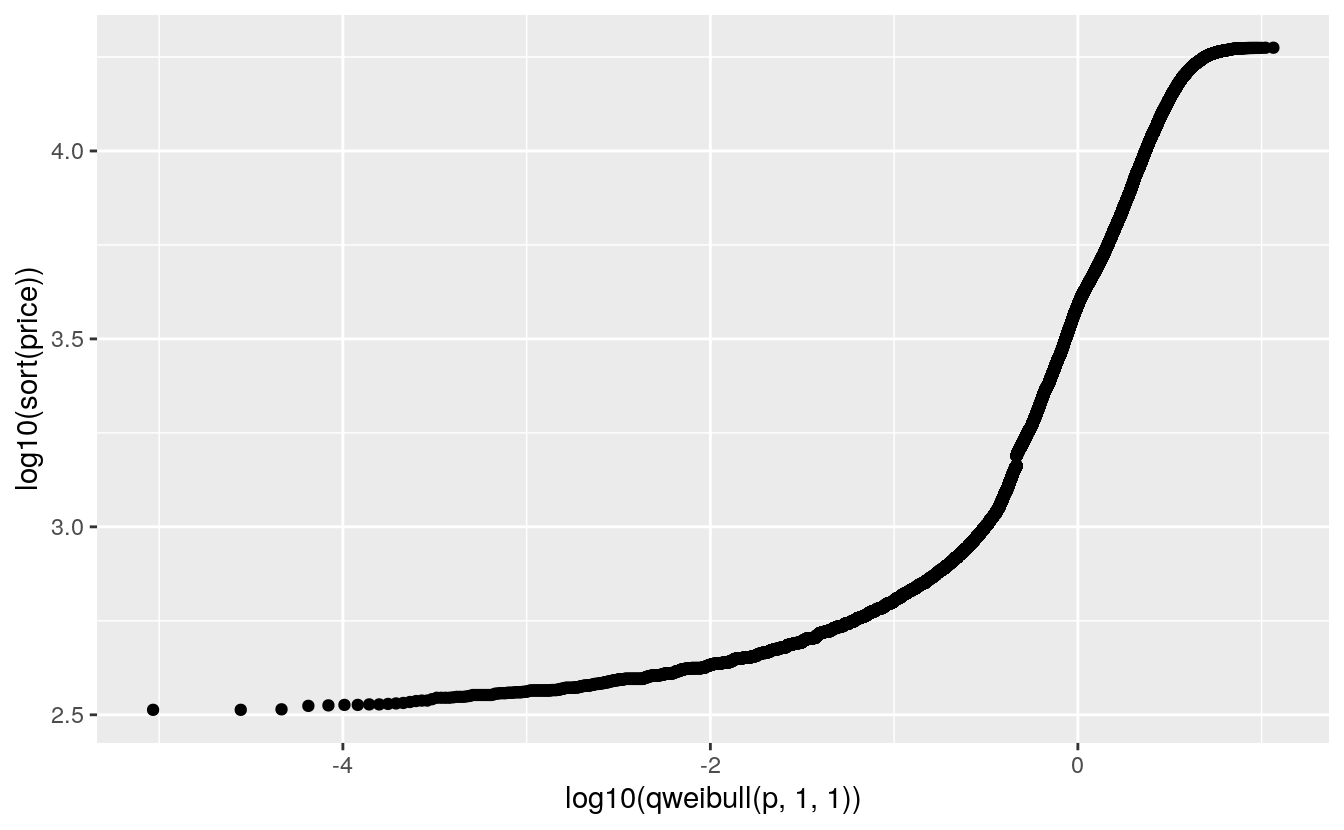• The lower tail does not match a Weibull distribution.

• Is this important?

• In engineering applications it often is.

• In selecting a reasonable model to capture the shape of this distribution it may not be.

• QQ plots are helpful for understanding departures from a theoretical model.

• No data will fit a theoretical model perfectly.

• Case-specific judgment is needed to decide whether departures are important.

• George Box: All models are wrong but some are useful.